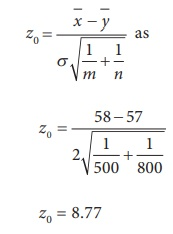Home | | Statistics 12th Std | Test of Hypotheses for Equality of Means of two Populations (Population Variances are Known)

# Test of Hypotheses for Equality of Means of two Populations (Population Variances are Known)

General Procedure for Test of Hypotheses for Equality of Means of two Populations (Population Variances are Known): Procedure Steps, Example Solved Problems

TEST OF HYPOTHESES FOR EQUALITY OF MEANS OF TWO POPULATIONS (Population variances are known)

### Procedure:

Step-1 : Let µX and σX2 be respectively the mean and the variance of Population -1. Also, let µY and σY2 be respectively the mean and the variance of Population -2 under study. Here σX2 and σY2 are known admissible values.

Frame the null hypothesis as H0: µX = µY and choose the suitable alternative hypothesis from

H1: µX ≠ µY (ii) H1: µX> µY (iii) H1: µX< µY

Step-2 : Let (X1, X2, …, Xm) be a random sample of m observations drawn from Population-1 and (Y1, Y2, …, Yn) be a random sample of n observations drawn from Population-2, where m and n are large(i.e., m ≥ 30 and n ≥ 30). Further, these two samples are assumed to be independent.

Step-3 : Specify the level of significance, α.

Step-4 : Consider the test statisticunder H0, whereandare respectively the means of the two samples described in Step-2.

The approximate sampling distribution of the test statistic Z =under H0 (i.e., µX = µY) is the N(0,1) distribution.

It may be noted that the test statistic, when.

Step-5 : Calculate the value of Z for the given samples (x1, x2, x3,…. xm) and (y1, y 2, y 3,…. ym) as.

Here,andare respectively the values ofandfor the given samples.

Step-6 : Find the critical value, ze, corresponding to α and H1 from the following tableStep-7 : Make decision on H0 choosing the suitable rejection rule from the following table corresponding to H1. corresponding to H1.### Example 1.10

Performance of students of X Standard in a national level talent search examination was studied. The scores secured by randomly selected students from two districts, viz., D1 and D2 of a State were analyzed. The number of students randomly selected from D1 and D2 are respectively 500 and 800. Average scores secured by the students selected from D1 and D2 are respectively 58 and 57. Can the samples be regarded as drawn from the identical populations having common standard deviation 2? Test at 5% level of significance.

### Solution:

Step 1 : Let μX and μY be respectively the mean scores secured in the national level talent search examination by all the students from the districts D 1 and D2 considered for the study. It is given that the populations of the scores of the students of these districts have the common standard deviation σ = 2. The null and alternative hypotheses are

Null hypothesis: H0: µX = µY

i.e., average scores secured by the students from the study districts are not significantly different.

Alternative hypothesis: H1: µX ≠ µY

i.e., average scores secured by the students from the study districts are significantly different. It is a two-sided alternative.

Step 2 : Data

The given sample information are

Size of the Sample-1 (m) = 500

Size of the Sample-2 (n) = 800. Hence, both the samples are large.

Mean of Sample-1 () = 58

Mean of Sample-2 () = 57

Step 3 : Level of significance

α= 5%

Step 4 : Test statistic

The test statistic under the null hypothesis H0 isSince both m and n are large, the sampling distribution of Z under H0 is the N(0, 1) distribution.

Step 5 : Calculation of Test Statistic

The value of Z is calculated for the given sample information fromStep-6 : Critical value

Since H1 is a two-sided alternative hypothesis, the critical value at α = 0.05 is ze = z0.025 = 1.96.

Step-7 : Decision

Since H1 is a two-sided alternative, elements of the critical region are defined by the rejection rule |z0 | ≥ z e = z0.025. For the given sample information, |z0| = 8.77 > ze = 1.96. It indicates that the given sample contains sufficient evidence to reject H0. Thus, it may be decided that H0 is rejected. Therefore, the average performance of the students in the districts D1 and D2 in the national level talent search examination are significantly different. Thus the given samples are not drawn from identical populations.

Tags : Procedure Steps, Example Solved Problems | Statistics , 12th Statistics : Chapter 1 : Tests of Significance - Basic Concepts and Large Sample Tests
Study Material, Lecturing Notes, Assignment, Reference, Wiki description explanation, brief detail
12th Statistics : Chapter 1 : Tests of Significance - Basic Concepts and Large Sample Tests : Test of Hypotheses for Equality of Means of two Populations (Population Variances are Known) | Procedure Steps, Example Solved Problems | Statistics